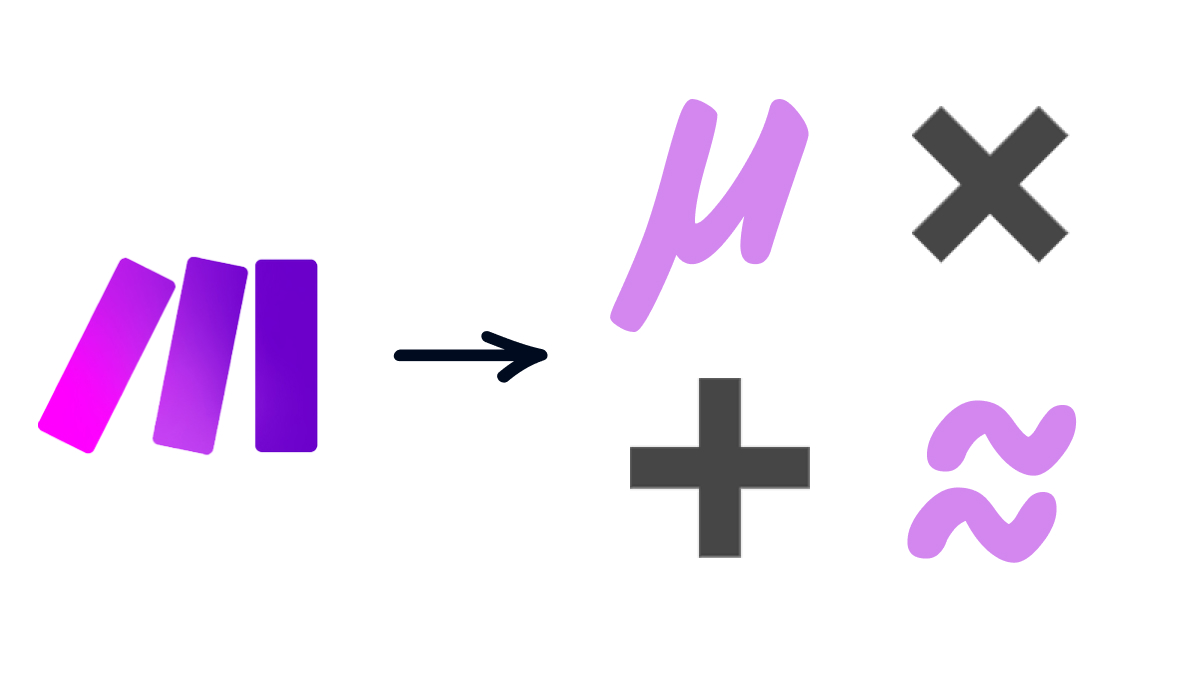# Run calculations in your Make scenarios with Math Functions

Make's wide variety of math functions allow you to add numbers together, round numbers to the nearest integer, calculate an average, and more. In this post, we'll show you how to use math functions in Make (formerly Integromat).

Written by
Matt Jasinski
and

December 14, 2022A few simple calculations can be the difference between a perfect workflow automation and a frustrating tool that doesn’t do what you need it to.

Make (formerly Integromat) gives you an extensive selection of math operations that you can use in any text field in your scenarios.

You can use these functions to add numbers, round off a decimal point, find the average value in a set of numbers, or even generate a random value.

In this post, we’ll show you how to perform each of these functions with short, step-by-step tutorials. We’ll use a Slack module as an example, so the result of every function will be sent as a Slack message.

With the “sum” function, Make will calculate the sum of every number you enter between the parentheses. Here’s how it works:

• Open any text field in your scenario

• Select the X1 symbol to access math functions

• Click on the ‘sum’ function to add it to your selected field

• Enter the numbers you want to add together, separated by semicolons

• Alternatively, you can enter an array of numbers to add together

• Run the module to output the sum

# Round decimal values to whole numbers

Make’s “round” function will round any number with a decimal point to the nearest whole integer. For example, 3.7 would be rounded to 4, while 7.23 would be rounded to 7. To use the round function:

• Open any text field in your scenario

• Select the X1 symbol to access math functions

• Click on the “round” function to add it to your selected field

• Enter the number you want to round between the parentheses

• Run the module, and the output will include the number rounded to the nearest integer

# Find the average of a set of numbers

Make lets you easily calculate the average of several numbers with this simple function. Just enter an array of numbers or values separated by semicolons to calculate the mean. For example, the average of 5, 6, and 7 would be 6.

• Open any text field in your scenario

• Select the X1 symbol to access math functions

• Click on the “average” function to add it to your selected field

• Enter the numbers that you want to use, separated by a semicolon

• Alternatively, you can enter an array of numbers

• Run the module to output the average

# Generating a random number

Generating a random number can be a useful technique for many automated workflows. To create one in Make, all you have to do is use the prebuilt variable called “random”. Here’s how it works:

• Open any text field in your scenario

• Select the X1 symbol to access math functions

• Click on the “random” variable to add it to your selected field

• Run the module to generate a random number between 0 and 1

### Generating a number between 0 and 100

In many cases, it will be more appropriate to generate a whole number between 0 and 100 rather than a decimal value between 0 and 1. To do that, you just need to multiply the “random” variable by 100 and round the result.

• Open any text field in your scenario

• Select the X1 symbol to access math functions

• Click on the “round” function to add it to your selected field

• Add the “random” variable between the parentheses of the “round” function

• Click on the green multiplication symbol to multiply the “random” variable by 100

• Run the module to check your result

## Build more advanced scenarios with math functions

Using Make’s math functions will let you build more sophisticated automations that transform your data and respond effectively to different circumstances. The functions in this post are just a sample of what’s available; try exploring the rest to discover everything you can do with these tools.